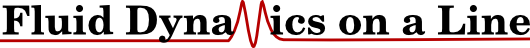# Teaching

This website includes slides, tutorial sheets and solutions for the various couses I teach at Imperial College London.

##A Complete 1D Navier-Stokes Solver on one page. A work in progress, a Jupyter notebook to explain the complete discretisation of the Navier Stokes equations in 1D, explaining the simplest possible case (1D) how we can discretise our equations, issues with osciallations, (eventually) boundary conditions and the fractional step pressure solver.

## Multi-Scale Modelling

Here are the notes for the continuum part of the multi-scale modelling course I teach. This is for masters students who have a background in a mathematical subject. Slides for the lectures, part one notes and part two notes two, as well as background notes.

The lectures are available:

• Part one video, introduction to the continuum, differential equations and numerical solutions.
• Part two video, review of part one, more differential equations and an overview of the steps which lead the the Navier-Stokes equation.
• A white-board derivation video of the Navier-Stokes equation considering the link to molecular systems.
The content includes:

• Where the continuum fits into the wider modelling hierarchy
• Understand the Continuum assumption
• Partial differential equations and numerical solutions
• Two dimensional vector fields
• The Navier-Stokes Equation
• Assumptions that lead to it
• Key terms and their meaning (with some extensions)
• Simplifications and solutions
• Link to the molecular dynamics equations
• Numerical solutions to the Navier Stokes equation

and the aims for the course were as follows:

• State the Continuum assumption, specifically for continuous fields and how this underpins fluid dynamics
• Understand three dimensional fields, vector calculus and partial differential equations
• Be able to solve basic differential equations numerically
• State the Navier-Stokes Equation, key assumptions, the meaning of the terms and how to simplify and solve.
• Understand how to treat the various terms in a numerical solutions to the Navier-Stokes equation
• Understand where the continuum modelling fits into the hierarchy and links to the molecular and plant scales

## Python

#### Intro Course

In order to address the lack of general Python teaching here at Imperial, I put together and gave a three part introduction course through the HPC support here at Imperial. This class was aimed at beginners and also for those who want to switch from Matlab to Python.

• Introduction to Python for scientific computing, 3/3/17 (Video) (Slides) (Solutions)
• Motivation for using Python.
• Introduction to programming in Python
• Python concepts (lists, iterators, etc) and discussion of the differences to other languages.
• Scientific libraries numpy and matplotlib.
• Examples of usage for scientific problems.
• Further details of the Python language, 10/3/17 (Video) (Slides) (Solutions)
• More on Python data structures: concepts like references, immutable, lists, data organisation with Dictionaries and numpy arrays.
• Use of functions and design of interfaces.
• Introduction to classes and objects.
• Structuring a project, importing modules and writing tests.
• Examples of usage for scientific problems.
• Python libraries, 17/3/17 (Video) (Slides) (Solutions)
• Using Python to read files (ascii, binary, hp5) and plot.
• Running parameter studies by calling executables repeatedly with subprocess.
• Designing a basic Graphical User Interface.
• Unit testing frameworks and version control.
• Other libraries and how to wrap your own code from fortran, c++, etc

Feedback from the course was very positive, summarised here, although given the large volume of material and range of students' backgrounds, many students felt the pace was too fast.

#### HPC Summer School 2017

Given the interest in this course, I ran again as part of the summerschool. This was split over two days:

Feedback from the course was positive, summarised here. The modular and object oriented approach taught in these course is the basis for open-source visualisation software, pydataview.

#### Roll Royce 2017

I was employed by Roll Royce to deliver a course over two days at their headquarters in Darsbury back in 2017. The feedback was very positive from this course, with 35 candidates rating the "professional competence of the trainer" as 2.89 out of 3, a rating of 2.7 out of 3 for "how satisfied were you with the organisation of the training" and an "overall rating for the course" of 2.7 out of 3. The full summary is here. Please contact me if out would be interested in organising teaching.